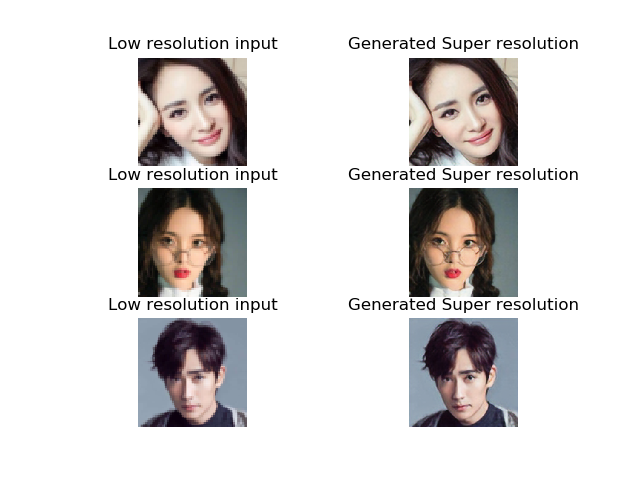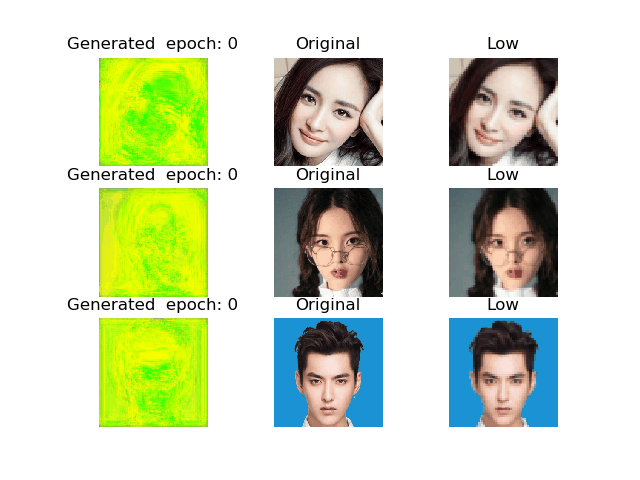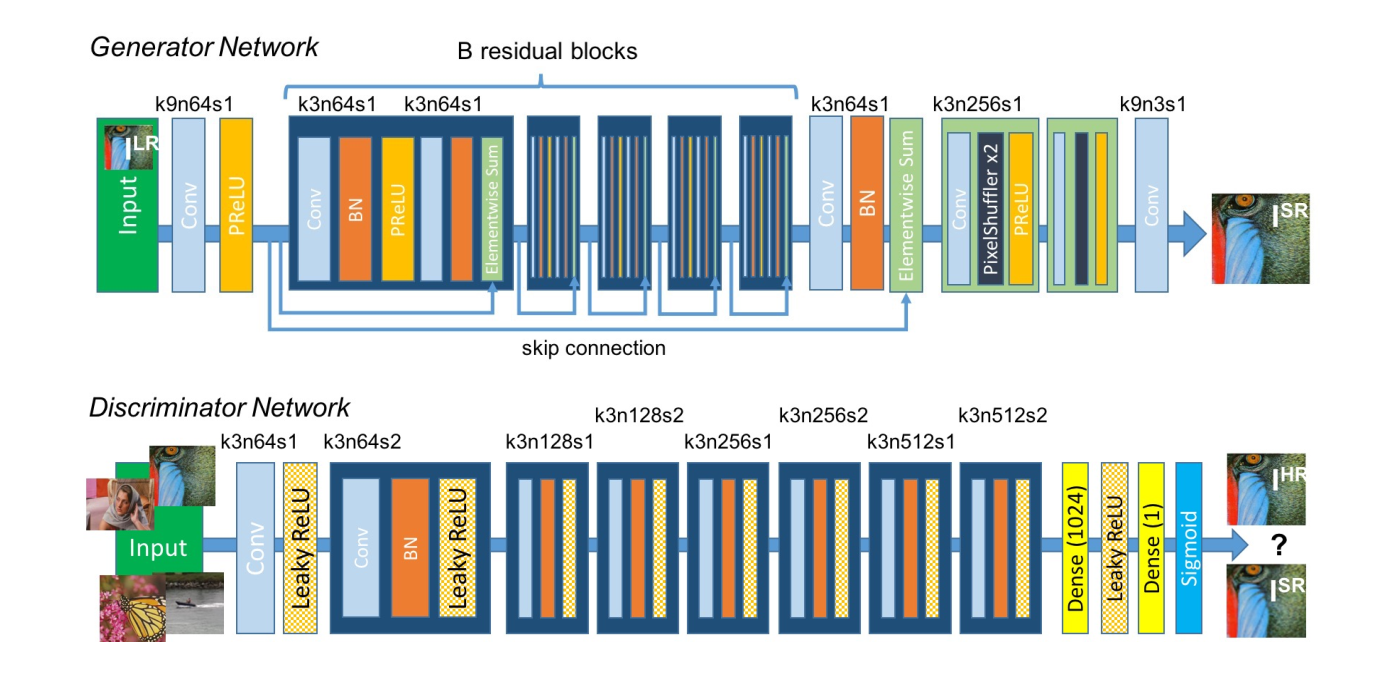# Demo效果# 原理分析

## 系统模型$\begin{array}{c}{\min _{\theta_{G}} \max _{\theta_{D}} \mathbb{E}_{I^{H R} \sim p_{\mathrm{ranin}}\left(I^{H R}\right)}\left[\log D_{\theta_{D}}\left(I^{H R}\right)\right]+} {\mathbb{E}_{I^{L R} \sim p_{G}\left(I^{L R}\right)}\left[\log \left(1-D_{\theta_{D}}\left(G_{\theta_{G}}\left(I^{L R}\right)\right)\right]\right.}\end{array}$

1. 固定生成器G (参数为${\theta_{G}}$), 训练判别器，使得其能够分辨 真实的图片和生成器生成的图片。
2. 固定判别器， 训练生成器， 使得其能够让固定的判别器将其判别为真实的图片
如此迭代之后， 判别器再也无法分别真实和生成的图片，此时就说明生成器生成的图片已经足够以假乱真。这里真实的图片指的就是高精度的图片，训练完成后，我们期望生成器可以接收低精度图片，来生成高精度图片。

## 损失函数

$\begin{array}{r}{l_{V G G / i . j}^{S R}=\frac{1}{W_{i, j} H_{i, j}} \sum_{x=1}^{W_{i, j} H_{i, j}}\left(\phi_{i, j}\left(I^{H R}\right)_{x, y}\right.} {-\phi_{i, j}\left(G_{\theta_{G}}\left(I^{L R}\right)\right)_{x, y} )^{2}}\end{array}$

$l^{S R}=\underbrace{l_{\mathrm{X}}^{S R}}_{\text { content loss }}+\underbrace{10^{-3} l_{G e n}^{S R}}_{\text { adversarial loss }}$

# 结束